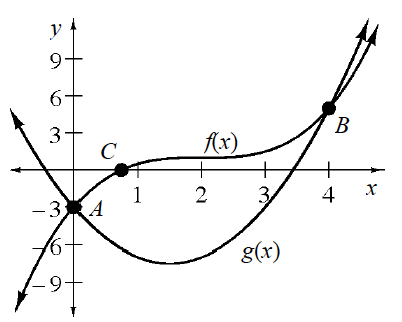### Home > INT3 > Chapter 3 > Lesson 3.1.2 > Problem3-39

3-39.

Consider the graph of $f(x)=\frac{1}{2}(x-2)^3+1$ and $g(x)=2x^2-6x-3$ at right.

1. Write an equation that you could solve using points $A$ and $B$. What are the solutions to your equation? Substitute them into your equation to show that they work.

Set $f(x)$ and $g(x)$ equal to each other.

$\frac{1}{2}(\textit{x}-2)^{3}+1=2\textit{x}^{2}-6\textit{x}-3$

2. Are there any solutions to your equation in part (a) that do not appear on the graph? Explain.

Solve the equation for $x$.

$\frac{1}{2}\left(\textit{x}^{3}-6\textit{x}^{2}+12\textit{x}-8\right)-2\textit{x}^{2}+6\textit{x}+4=0$

$\frac{1}{2}\textit{x}^{3}-5\textit{x}^{2}+12\textit{x}=0$

$\textit{x}\left(\frac{1}{2}\textit{x}-3\right)(\textit{x}-4)=0$

3. Write an equation that you could use to solve for the $x$-coordinate of point $C$. Estimate the solution to your equation using the graph. Again, substitute your solution into your equation. How close was your estimate?

Set $f(x)$ equal to $0$.